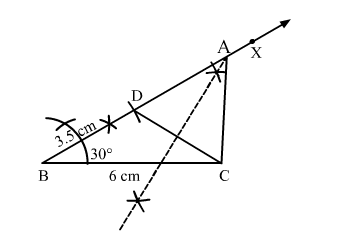# Construct a ∆ABC in which BC = 6 cm, ∠B = 30° and AB – AC = 3.5 cm.

Question:

Construct a ∆ABC in which BC = 6 cm, ∠= 30° and AB – AC = 3.5 cm. Justify your construction.

Solution:Steps of construction:
1. Draw a line segment BC = 6 cm.

2. Construct $\angle C B X=30^{\circ}$

3. Set off BD = 3.5 cm.
4. Join DC.
5. Draw the right bisector of DC, meeting BD produced at A.
6. Join AC.

Thus, $\triangle A B C$ is the required triangle.

Justification:
Point A lies on the perpendicular bisector of DC.